In :
%matplotlib inline
import numpy, scipy, matplotlib.pyplot as plt, IPython.display as ipd
import librosa, librosa.display
import stanford_mir; stanford_mir.init()


# Zero Crossing Rate¶

The zero crossing rate indicates the number of times that a signal crosses the horizontal axis.

In :
x, sr = librosa.load('audio/simple_loop.wav')


Listen to the signal:

In :
ipd.Audio(x, rate=sr)

Out:

Plot the signal:

In :
plt.figure(figsize=(14, 5))
librosa.display.waveplot(x, sr=sr)

Out:
<matplotlib.collections.PolyCollection at 0x10a3e1ac8>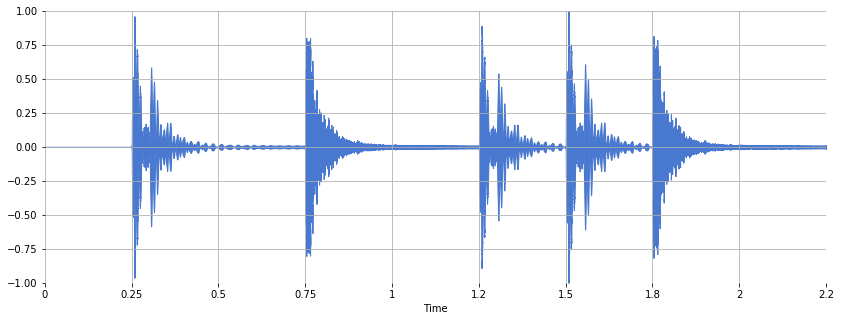Let's zoom in:

In :
n0 = 6500
n1 = 7500
plt.figure(figsize=(14, 5))
plt.plot(x[n0:n1])

Out:
[<matplotlib.lines.Line2D at 0x10a3893c8>]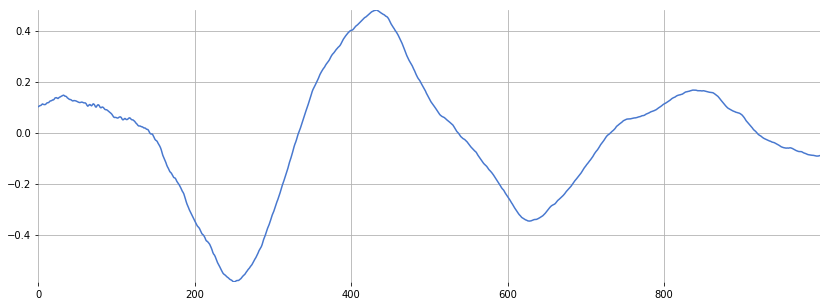I count five zero crossings. Let's compute the zero crossings using librosa.

In :
zero_crossings = librosa.zero_crossings(x[n0:n1], pad=False)

In :
zero_crossings.shape

Out:
(1000,)

That computed a binary mask where True indicates the presence of a zero crossing. To find the total number of zero crossings, use sum:

In :
print(sum(zero_crossings))

5


To find the zero-crossing rate over time, use zero_crossing_rate:

In :
zcrs = librosa.feature.zero_crossing_rate(x)
print(zcrs.shape)

(1, 97)


Plot the zero-crossing rate:

In :
plt.figure(figsize=(14, 5))
plt.plot(zcrs)

Out:
[<matplotlib.lines.Line2D at 0x10a4e0ba8>]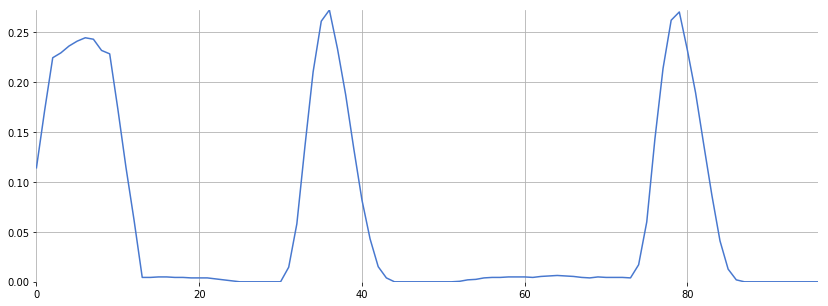Note how the high zero-crossing rate corresponds to the presence of the snare drum.

The reason for the high rate near the beginning is because the silence oscillates quietly around zero:

In :
plt.figure(figsize=(14, 5))
plt.plot(x[:1000])
plt.ylim(-0.0001, 0.0001)

Out:
(-0.0001, 0.0001)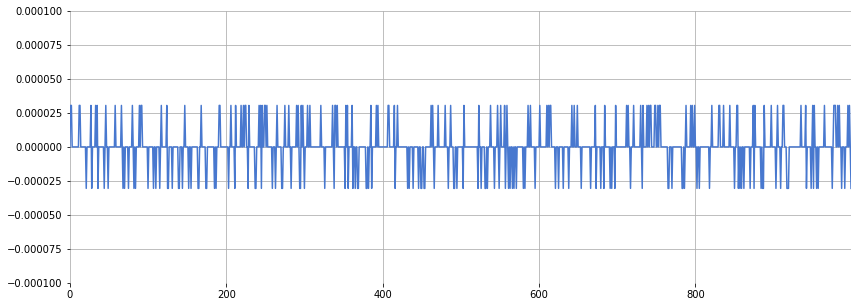A simple hack around this is to add a small constant before computing the zero crossing rate:

In :
zcrs = librosa.feature.zero_crossing_rate(x + 0.0001)
plt.figure(figsize=(14, 5))
plt.plot(zcrs)

Out:
[<matplotlib.lines.Line2D at 0x10a4510f0>]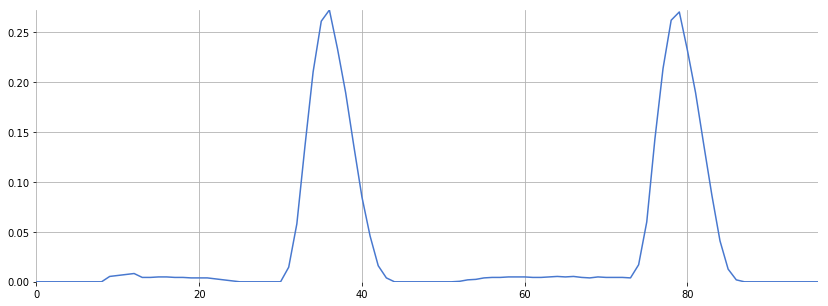## Questions¶

Try for other audio files. Does the zero-crossing rate still return something useful in polyphonic mixtures?

In :
ls audio

125_bounce.wav                  jangle_pop.mp3
58bpm.wav                       latin_groove.mp3